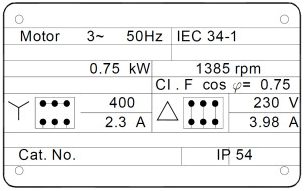# ST500 | Initial operation – What should I watch for (motor data)?

Before a initial operation of a frequency inverter you should absolutely check that the factory settings are loaded y0.00 = 3. Then the motor rated data of the used motor must be programmed into the inverter. This motor data can be found on the nameplate of the motor.

Example of a nameplate which is part of a three-phase asynchronous motor:Figure: Nameplate

The required parameters are located in the -b- parameter group. These are the parameters from b0.00 to b0.14.

• Nominal voltage (b0.02): 230V (delta) / 400V (star)
• Nominal frequency (b0.04): 50Hz (set at more than 50Hz F0.19 first)
• Nominal current (b0.03):3,98A (delta) / 2,30A (star)
• Nominal speed (b0.05):1385 R/min
• Nominal power (b0.01):0,75kW
• All other parameters can be determined independently by measurement by the inverter. For this b0.27 = 1 set for the rotating measurement without load and b0.27 = 2 for the stationary measurement with load. If possible, always lead by a rotating measurement without load.

Back to the ST500 frequency inverter questions.

Tags: